# Capacitance transducer types, applications and characteristics

Capacitance transducers are passive transducers that work on the principle of change of capacitance. Here we will discuss the principle, types, applications and advantages of the capacitance transducers.

## Design of a basic capacitance transducer

A capacitor consists of two parallel conducting plates of area A placed at a distance d from each other with any material (insulator) between them. The dielectric constant ε is unique for different medium

## Principle of a basic capacitance transducer

Capacitance is defined as the function of the geometric quantities, such as the area of the conducting plate and the distance between them, and the permittivity of the dielectric medium between them.

Where ε is the dielectric constant, permittivity, of the dielectric medium. A is the area of the electrode, more specifically overlapping area. d is the distance between the two parallel electrodes Capacitance can change with change in any one of the above parameters. For a capacitor with an insulating material separating the conducting plates, the capacitance is given as

Where,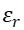is the dielectric constant of insulating medium. For air=1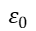is the permittivity of air or free space (8.85 X 10-12F/m) In a capacitance transducer, the change in the overlapping area and the distance between the plates is attributed to the movement due to external force, typically used to sense displacement, pressure, force. The change in dielectric medium is often attributed to the displacement of the original medium which is often used in level measurement for fluids. Depending on the design of the capacitor there is change in the sensitivity which plays a major role in the application .

## Types of capacitance transducers

** 1. Parallel plate capacitance with Rectangular plates**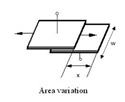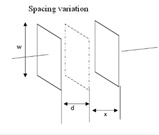Here one of the plates is fixed while the other is movable, depending on the nature of the movement, there may be variation in the area A or the distance d. The change in capacitance is given as, In case of area variation, where the capacitance plate moves a distance of x,

In case of spatial variation, where the distance between the plates increase by a x,

Sensitivity of the Parallel plate capacitance transducer: Sensitivity of the transducer is given as the change in the output to the change in input. In this case sensitivity S is dc/dx Area variation: Distance variation:

2. Cylindrical capacitor transducer A cylindrical capacitor is two concentric cylinders with a dielectric in between.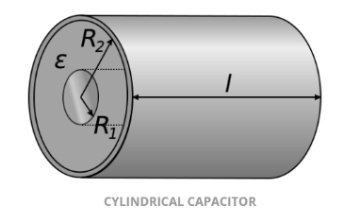The surface area of the cylinder of radius x meters and unit length is given as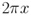The electrical field intensity at a point x meters away from the centre of cylinder is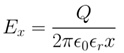The potential difference developed between the cylinders is given as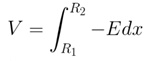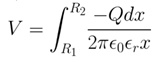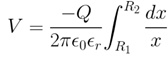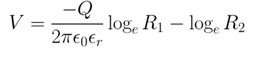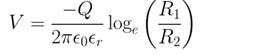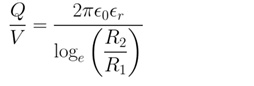In that case the capacitance is given as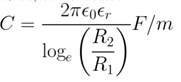Considering a length of the cylinders to be L,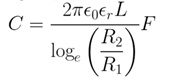Numerically substituting D instead of R will give the same result. Sensitivity in this case is S = dC/dL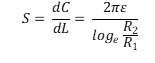3. Semi circular parallel plate Mostly used to measure the angular displacement, the capacitance is maximum when the plates completely overlap.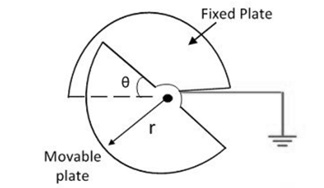Area of the plate is given as

Therefore, the capacitance is given by In case of an angular displacement of θ

180 degree is the maximum displacement of the capacitor.

Sensitivity is given by

4. Change of dielectric between parallel plates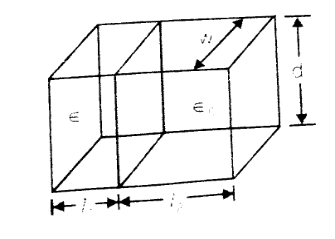The capacitance of the parallel plate with different dielectric is given as

This is the principle used in liquid level measurement.

5. Change in distance between circular plates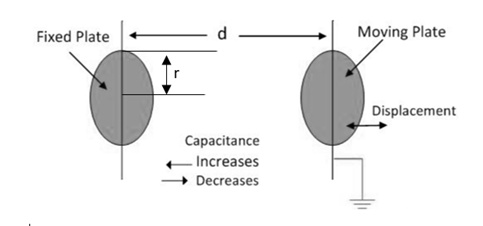The capacitance is given by:

When the plates move a distance of x And the sensitivity is given as

### Output characteristics of a capacitor transducer

With distance between the plates d The relation between the input d and output C are in the powers of 2 and inversely proportional. The output is not linear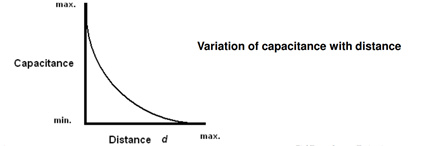With change in area A due to displacement The is a linear relationship between the input and output.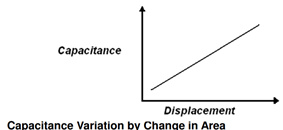With Angular displacement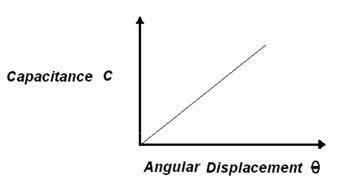a. Sensitive is very high
b. High frequency response
d. Very good resolution

a. Non linear behaviour due to edge effect
b. Temperature sensitive
c. Affected by stray capacitance

Capacitive transducers have the dynamic characteristics similar to high pass filter

## Applications of capacitive transducer

Used in pressure, displacement, force and level measurement.

Special capacitance transducers:

Variable capacitance pressure transducer Containing a diaphragm instead of one of the fixed plates, the dielectric material is air. The flexible diaphragm is pulled taut but bends into a curve on pressure.

For any radius r, the deflection y is given as Where a = radius of the diaphragm t = Thickness of the diaphragm E = Young’s modulus v = Poisson’s ratio The increase in capacitance is given by :

C is the capacitance at zero pressure which is This is the same principle used in capacitor microphone which converts electrical signal to sound signal.

High precision differential capacitor This uses a three plate differential ( push-pull displacement) sensor consisiting of a movable plate M in between two fixed plates P 1 and 2. X is the displacement of the movable plate, the capacitances is given by,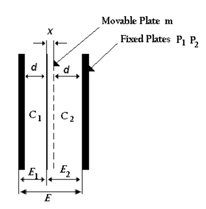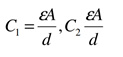The voltages across C1 and C2 is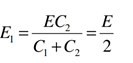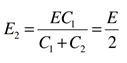At midpoint , E1 – E2 = 0 With x being the displacement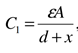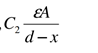The differential output is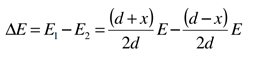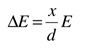The sensitivity is given as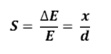The output varies linearly and gives an accuracy of 0.05 %

1 Like